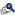Modified exact poly-3 testView Presentation Karl K Lin, FDA  *Min Min, FDA  Atiar Mohammad Rahman, FDA  Keywords: ratio estimate, p-value, proc stratify, permutation Preclinical animal carcinogenicity studies are usually concerned with testing the statistical significance of a dose-response relationship. The Age-adjusted exact trend test (J. Y. Mancuso, H. Ahn, J. Chen and J. P. Mancuso, 2002, Biometrics 58, 403-412) is proposed to adjust for the presence of higher mortality rates with increasing dose which is usual in practice. The proposed test can be regarded as an exact version of the poly-3 test (Bailer and Portier, 1988, Biometrics 44, 417-431). PROC STRATIFY (in STATXACT7) computing formula derives from the assumption that the row and column sums in the contingency tables are fixed. However, when time-at-risk weights are introduced to correct for treatment-related differences in survival, the denominators of the quantal response rates are subject to random variation. Ratio estimate (Bieler and Willaims, 1993, Biometrics 49, 793-801) uses the delta method of estimating the variance of ratio statistics derived from animal carcinogenicity experiments. This talk will be focused on investigations of p-value calculations based on difference computing methods. There are two p-value calculation comparisons: 1) between Proc stratify and exact ratio estimate 2) between Proc stratify and asymptotic ratio estimate The simulation results show that there is a good agreement between Proc stratify and exact ratio estimate methods. The power calculations in exact ratio estimate method will be included.